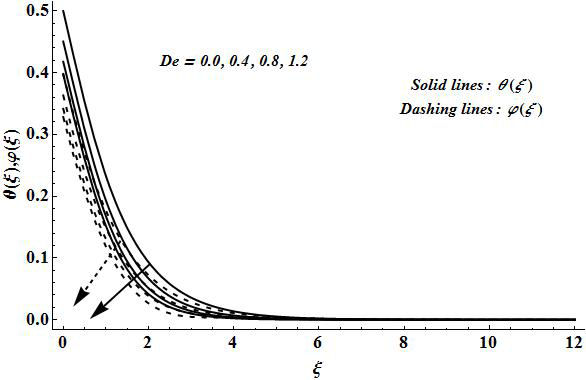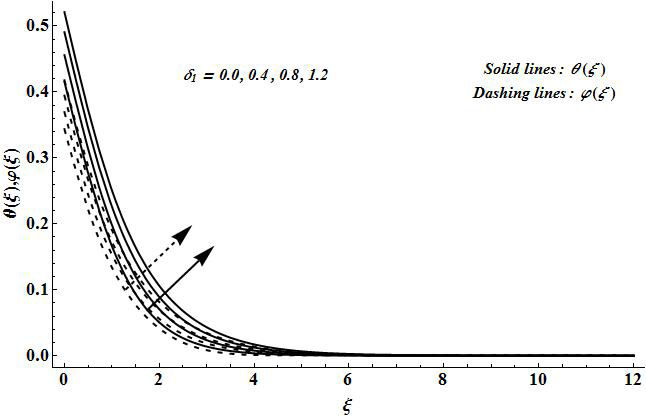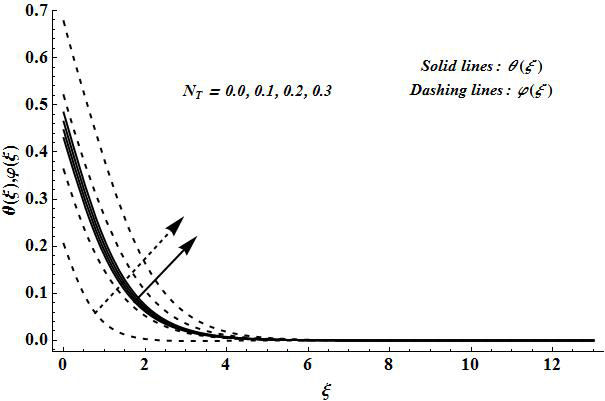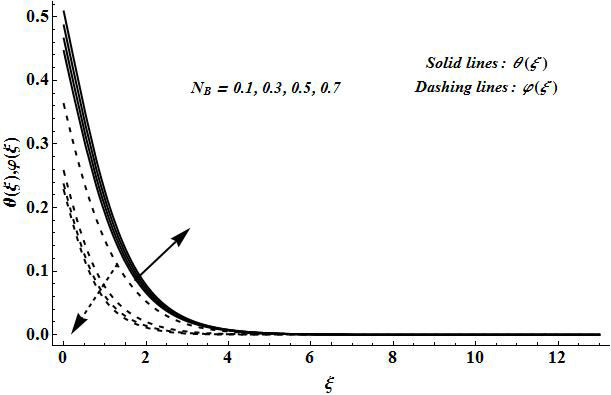## Artigo

•Citado por SciELO
•Acessos

•Similares em SciELO

## versão impressa ISSN 0035-001X

### Rev. mex. fis. vol.64 no.6 México Nov./Dez. 2018  Epub 05-Nov-2019

#### http://dx.doi.org/10.31349/revmexfis.64.628

Research

Magnetohydrodynamic Jeffrey nanoliquid flow with thermally radiative Newtonian heat and mass species

Abastract

This study characterizes the properties of Newtonian heat and mass species conditions in three-dimensional Jeffrey nanoliquid flow generated by the movement of thermally radiative surface. The liquid flow is electrically conducting through the consideration of magnetic field. The aspects of heat absorption, generation, and thermal radiation are considered in the equation of energy conservation. The boundary layer phenomenon is employed to obtain the mathematical expressions of considered physical model. These equations are solved via homotopic scheme. The convergence of homotopic solutions is validated by the numerical data. The importance of physical constraints on temperature, and nanoparticle concentration of liquid is visualized by the graphical results.

Keywords: Three-dimensional flow; Jeffrey liquid; nanoparticles; Newtonian conditions; bidirectional surface

PACS: 65.80.-g; 82.60.Qr

1. Introduction

Complex liquids have common nature of viscoelasticity. Some examples of complex viscoelastic liquids include suspensions of colloidal, polymer solutions, and polymer melts. Such fluids are generally involved in blow, and injection molding, extrusions, lab on a chip, inkjet printers, and many others. Viscoelastic behavior of liquids may affect the different aspects of flows like transport, and energy dissipation, hydraulic resistance, flow stability and mixing performance . Intra and inter molecules reactions of polymer chains may generate the viscoelasticity. Analysis and modeling of viscoelastic fluid flows have technical and scientific interests. Researchers of modern world have paid full attention to elaborate the mechanism of viscoelastic materials for better quality in industrial manufacturing of products. Many complex rheological expressions of viscoelastic materials had been developed in the past. A very fabulous model of viscoelastic liquids is known as Jeffrey fluid. This model has the features of stress retardation, and relaxation. Various investigators have adopted this model to discuss the different problems of fluid flows under multiple situations. For example, Turkyilmazoglu and Pop  modeled the flow of Jeffrey fluid due to shrinking or stretching of surface and express the exact behavior of govern mathematical expressions. Das et al. disclosed the nature of thermal magnetic field in stretched Jeffrey liquid flow with transport of melting heat. Gravitational aspects on Jeffrey liquid flow with solutal and thermal stratification have been reported by Abbasi et al.. Hayat et al. addressed the convective heating effects in hydromagnetic stagnant flow of Jeffrey fluid. Qayyum et al.  discussed the reactive Jeffrey fluid flow over variable thickness surface.

Nanofluids have achieved special attention due to their broad applications in modern science, and technology like glass fiber, electronic chips, micro-computers, wire drawing, renewable energy sources, engine oils, vehicles, systems of thermal energy storage, and many others. Some common base liquids such as oil, grease, water, and ethylene glycol are poor conductors and have lesser thermal efficiency. Researchers have developed modern technique to augment the thermal performance of such liquids with insertion of nanoparticles. The involvement of solid particles may alter the density, viscosity, thermal conductivity, and heat capacity of such liquids. In nanofluids mechanisms, there are various slip factors include thermophoretic, and Brownian movement aspects which are responsible to motion of nanoparticles in base liquids. Concentration of nanoparticles may not be constant due to presence of velocity slip factor of nanoliquids. Investigators of the modern era have proven that nanoparticles have an ability of transport energy, and also alter the thermophysical features. Buongiorno  was the first who explored the forces which have impacts on nanoparticles velocity. He reported that Brownian movement and thermophoretic are the forces which generate drift-flux in nanoparticles. After that, different attempts have been made by using the Buongiorno model to explore the mechanism of such factors under various circumstances and conditions [8-20].

Here our attention is to discuss the nature of Newtonian heat and mass transportation in three-dimensional magnetohydrodynamic flow of Jeffrey nanoliquid induced by the bidirectional movement of surface. In the past, the investigators discuss the various fluid flows problems by only considering the Newtonian heating aspects. They ignored the Newtonian mass transport effects. In practical situations, heat and mass transport occur simultaneously and if Newtonian heat phenomenon takes place, then the importance of Newtonian mass cannot be ignored. Here we considered both Newtonian heat and mass conditions because the problems of modern industrial processes. This phenomenon is more realistic,and practical. We also considered the solar radiation term in energy expression. All the governing equations are tackled by homotopic procedure [21-26]. The results are plotted for different values of physical constraints.

2. Governing problems

We assumed the three-dimensional steady-state flow of Jeffrey liquid induced by the movement of thermally radiative surface (Fig. 1). The impacts of heat absorption, generation, and thermal radiation are accounted in the expressions of energy. The Newtonian heat and mass species conditions are imposed at boundary of surface. The equations of considered flow phenomenon through boundary layer theory are

ux+vy+wz=0, (1)

uux+vuy+wwz=υ1+δ1(2uz2+δ2×(uz2vxz+vz2vyz+wz2vz2+u3uxz2+v3uyz2+w3uz3))-σB02ρfu, (2)

uux+vuy+wwz=υ1+δ1(2vz2+δ2×(uz2vxz+vz2vyz+wz2vz2+u3uxz2+v3uyz2+w3uz3))-σB02ρfv, (3)

uTx+vTy+wTz=2Tz2+τ(DBCzTz+DTT(Tz)2)+1(ρc)f16σ*T33k*2Tz2+Q(T-T), (4)

uCx+vCy+wCz=DB2Cz2+DTT2Tz2 (5)

where the respective velocity components in the x-, y- and z- directions are denoted by u, v and w, δ1 the ratio of relaxation to retardation times, δ2 the retardation time, T the fluid temperature, υ=(μ/ρ) the kinematic viscosity, μ the dynamic viscosity of fluid, ρf the density of fluid, σ the electrical conductivity, α the thermal diffusivity, Q>0 the heat generation parameter, and Q<0 represents the heat absorption parameter, τ=((ρc)p/(ρc)f) the ratio of nanoparticle heat capacity, and the base fluid heat capacity, σ* the Stefan-Boltzmann constant, k* the mean absorption coefficient, DB the Brownian diffusion coefficient, and DT the thermophoretic diffusion coefficient.

The boundary conditions can be written in the following forms:

u=Uw(x)=ax,v=Vw(y)=by,w=0,Tz=-htT,Cz=-hcC,atz=0 (6)

u0,v0,TT,CC,asz, (7)

where a and b are the constants of dimension time inverse, ht conjugate coefficient of heat transport, and hc conjugate coefficient of mass transport.

By employing the following variables

u=dxf'(ξ),v=dyg'(ξ),w=-dv(f'(ξ)+g(ξ)),ξ=zdυ,θ(ξ)=T-TT,ϕ(ξ)=C-CC, (8)

Eq. (1) is satisfied automatically, and Eqs. (2)-(7) can be converted in the following forms:

f+(1+δ1)((f+g)f-f'2)+De1(f2-(f+g)f-g'f)-(1+λ1)Mf'=0, (9)

g+(1+δ1)((f+g)g-g'2)+De1(g2-(f+g)g-f'g)-(1+λ1)Mg'=0, (10)

(1+43TR)θ+Pr(f+g)θ'+NBθ'φ'+NTθ'2+PrSθ=0, (11)

φ+PrLe(f+g)φ'+(NT/NB)θ=0, (12)

f=0,g=0,f'=1,g'=δ,θ'=-NH(1-θ(0)),ϕ'=-NC(1-ϕ(0))atη=0, (13)

f'0,g'0,θ0,ϕ0asη, (14)

where De=δ2d is the Deborah number, Mg=(σB02/dρf) the magnetic parameter, α=b/a is a ratio parameter, Pr=υ/α is the Prandtl number, TR=4σT3/kk* is the thermal radiation parameter, S=Q/dρcp is the internal heat generation parameter, NB=(τDB/υ) is the Brownian motion parameter, NT=(τDT/υT) is the thermophoresis parameter, NH=htυ/d and NC=hCυ/d are the Biot numbers, Le=α/DB is the Lewis number, and prime shows the differentiation with respect to ξ. The local Nusselt number NuRex-1/2 and Sherwood number ShRex-1/2 in dimensionless forms can be described as follows:

NuRex-1/2=NH(1+43TR)(1+1θ(0)),ShRex-1/2=NC(1+1φ(0)), (15)

in which Rex=uw(x)x/υ is the local Reynolds number.

3. Series solutions

The initial guesses and auxiliary linear operators for present case can be selected in the forms:

f0(ξ)=1-exp(-ξ),g0(ξ)=δ(1-exp(-ξ)), (16)

θ0(ξ)=NHexp(-ξ)1+NH,φ(ξ)=NCexp(-ξ)1+NC, (17)

Lf=f-f',Lg=g-g',Lθ=θ-θ,Lφ=φ-φ. (18)

We observe that the auxiliary linear operators may satisfy the following expressions

L(f)(E1+E2eξ+E3e-ξ)=0,L(g)(E1+E2eξ+E3e-ξ)=0,L(θ)(E7eξ+E8e-ξ)=0,L(φ)(E9eξ+E10e-ξ)=0,

where Ei(i=1-10) are the arbitrary constants.

4. Analysis of convergence and results

An analytical technique namely homotopy analysis scheme has been implemented to obtain the solutions of governing mathematical expressions. It is a well-established fact that convergence of homotopic solutions greatly depends on the proper choice of auxiliary parameters. We plotted curves at 15th order of deformations to select the reasonable values of auxiliary parameters f, g, θ and φ. For the presentproblem, the solutions should be convergent in the range of -0.85f-0.15, -0.90g-0.20, -1.20θ-0.15 and -1.00φ-0.25 (see Fig. 2). Table I executes that the present solutions converge from order of deformations. This Table is computed by choosing f=g=θ=φ=-65/100, De=0.4, δ1=0.3, Mg=0.7, Pr=1.5=Le, NB=0.1=NT, NH=0.2=NC, S=0.1, TR=0.3, and δ=0.5. Here we analyzed that the magnitude values of f(0) are larger in comparison to g(0), θ'(0) and φ(0).Figure 2 The - curves for f(ξ), g(ξ), θ(ξ) and φ(ξ) at 15th-order of deformations when De=0.4, δ1=0.3, Mg=0.7, Pr=1.5=Le, NB=0.1=NT, NH=0.2=NC, δ=0.5, S=0.1, and TR=0.3.

Table I Convergence of series solutions for different order of approximations when De=0.4,δ1=0.3,Mg=0.7,Pr=1.5=Le,NB=0.1=NT,NH=0.2=NC,S=0.1,TR=0.3,δ=0.5,and f=g=θ=φ=-65/100

Order of HAM approximations -f(0) -g(0) -θ'(0) -φ'(0)
01 1.26661 0.64143 0.26625 0.26777
07 1.26718 0.68497 0.28911 0.27261
12 1.26722 0.68482 0.29060 0.27274
18 1.26727 0.68480 0.29080 0.27279
23 1.26726 0.68489 0.29082 0.27278
28 1.26732 0.68504 0.29081 0.27277
35 1.26732 0.68504 0.29081 0.27277
40 1.26732 0.68504 0.29081 0.27277

Figures 3-10 are produced to elaborate the nature of emerging physical constraints on temperature θ(ξ) and nano-Figure 3 Curves of θ(ξ), and φ(ξ) for different values of De when δ1=0.3, Mg=0.7, Pr=1.5=Le, NB=0.1=NT, NH=0.2=NC, δ=0.5, S=0.1, and TR=0.3.Figure 4 Curves of θ(ξ), and φ(ξ) for different values of δ1 when De=0.4, Mg=0.7, Pr=1.5=Le, NB=0.1=NT, NH=0.2=NC, δ=0.5, S=0.1, and TR=0.3.Figure 5 Curves of θ(ξ), and φ(ξ) for different values of Mg when De=0.4, δ1=0.3, Pr=1.5=Le, NB=0.1=NT, NH=0.2=NC, δ=0.5, S=0.1, and TR=0.3.Figure 6 Curves of θ(ξ), and φ(ξ) for different values of Pr when De=0.4, δ1=0.3, Mg=0.7 Le=1.5, NB=0.1=NT, NH=0.2=NC, δ=0.5, S=0.1, and TR=0.3.Figure 7 Curves of θ(ξ), and φ(ξ) for different values of Le when De=0.4, δ1=0.3, Mg=0.7 Pr=1.5 NB=0.1=NT, NH=0.2=NC, δ=0.5, S=0.1, and TR=0.3.Figure 8 Curves of θ(ξ), and φ(ξ) for different values of NT when De=0.4, δ1=0.3, Mg=0.7 Pr=1.5=Le NB=0.1, NH=0.2=NC, δ=0.5, S=0.1, and TR=0.3.Figure 9 Curves of θ(ξ), and φ(ξ) for different values of NB when De=0.4, δ1=0.3, Mg=0.7 Pr=1.5=Le, NT=0.1, NH=0.2=NC, δ=0.5, S=0.1, and TR=0.3.Figure 10 Curves of θ(ξ), and φ(ξ) for different values of TR when De=0.4, δ1=0.3, Mg=0.7, Pr=1.5=Le, NB=0.1=NT, NH=0.2=NC, δ=0.5, S=0.1.

particle concentration φ(ξ). Figure 3 clearly describes that an increment in Deborah number De leads to smaller profiles of θ(ξ), and φ(ξ) Here De directly depends on stress retardation δ2. The stress retardation is stronger with an enhancement in De. Such stronger stress retardation creates a reduction in the profiles θ(ξ), and φ(ξ). The results in the case of fluid liquid can be achieved when De=0, and δ1=0. The features of ratio stress relaxation and stress retardation δ1 on θ(ξ) and φ(ξ) are investigated in Fig. 4. The curves of θ(ξ) and φ(ξ) are boost up when we use the larger δ1. From physical concept, the higher δ1 implies to larger stress relaxation in comparison to stress retardation. This fact leads to stronger temperature and nanoparticles concentration against the larger δ1. Figure 5 incorporates the impacts of magnetic parameter Mg on θ(ξ), and φ(ξ). This figure clearly executes that an increment in Mg give rise to the profiles of θ(ξ) and φ(ξ). Here the Lorentz force exists due to electrically conducting liquid. This Lorentz force becomes stronger for larger Mg that resists the flow of liquid. Such resistance in liquid flow leads to higher temperature, and nanoparticle concentration. Further, the hydrodynamic flow situation can be created by setting Mg=0. In case of hydrodynamic flow, the profiles of θ(ξ), and φ(ξ) are minimum position.

From Fig. 6, we evaluated the nature of Prandtl number Pr on θ(ξ), and φ(ξ). The increasing values of Pr correspond to weaker profiles of temperature, and nanoparticle concentration. The conductivity of liquid is lesser for higher Pr, and stronger for smaller Pr due to fact the profiles of θ(ξ), and φ(ξ) decay. The proper choice of Pr in manufacturing processes of industry is very essential because the suitable values of Pr are utilized to provide the best heat transport rate to achieve the highest quality product. The execution of Lewis number Le on θ(ξ), and φ(ξ) is presented in Fig. 7. This figure clearly elucidates that the variations in θ(ξ) are very lesser in comparison to φ(ξ) as we use the larger Le. Moreover, the curves of θ(ξ), and φ(ξ) are decreased for the increasing values of Le. The coefficient of Brownian diffusion becomes weaker for higher Le because it is inversely related to it. Such weaker Brownian coefficient factor leads to decrement in the profiles of θ(ξ), and φ(ξ).

The impact of parameter of thermophoretic Nt on θ(ξ), and φ(ξ) is discussed through Fig. 8. Figure 8 clearly depicts that profiles of both θ(ξ), and φ(ξ) are risen due to rising values of NT. The stronger NT corresponds to an enhancement in thermal conductivity of liquid due to consideration of nanoparticles. This higher thermal conductivity factor leads to higher θ(ξ), and φ(ξ). The change in θ(ξ) for various values of NT are significant as compared to φ(ξ). The increasing values of parameter of Brownian movement NB give risen to the profiles of θ(ξ), and φ(ξ) (see Fig. 9). The liquid temperature θ(ξ) is boost up by an increment in NB while the curves of φ(ξ) are retarded. In fact, NB is appeared in form of (1/NB) that corresponds to weaker nanoparticle concentration. The nature of parameter of thermal radiation TR on liquid temperature, and nanoparticle concentration is described in Fig. 10. Here we investigated that the presence of TR give risen to θ(ξ), and φ(ξ). The case of absence of radiative effect can be discussed when TR=0. The radiation factor gives more heat to liquid due to which temperature and concentration are enhanced.

5. Conclusions

This research work executes the features of Newtonian conditions in three-dimensional Jeffrey liquid flow under the impact of magnetic field, thermal radiation, and suspension of nanoparticles. We employed the boundary layer concept to govern the expressions of physical phenomenon. The convergent solutions are developed and plotted. We observed that the ratio of stress relaxation and stress retardation δ1, and Deborah number De have totally reverse influences on liquid temperature θ(ξ), and nanoparticle concentration ϕ(ξ). An increment in magnetic parameter Mg give risen to the profiles of θ(ξ), and φ(ξ). This phenomenon occurs due to involvement of Lorentz force. The change in profiles of θ(ξ) for various Pr is significant as compared to profile of temperature in case of various Le. The liquid temperature, and nanoparticle concentration enhanced by increasing the values of thermophoretic factor NT. An increase in factor of Brownian movement lead to higher temperature curves but lower curves of nanoparticle concentration. The involvement of radiation factor gradually enhanced the temperature of liquid.

References

1 R. Comminal, J.H. Hattel, M.A. Alves, and J. Spangenberg, Journal of Non-Newtonian Fluid Mechanics 237 (2016) 1-15. [ Links ]

2 M. Turkyilmazoglu, and I. Pop, International Journal of Heat and Mass Transfer 57 (2013) 82-88. [ Links ]

3 K. Das, N. Acharya, and P.K. Kundu, Alexandria Engineering Journal 54 (2015) 815-821. [ Links ]

4 F.M. Abbasi, S.A. Shehzad, T. Hayat, and M.S. Alhuthali, Journal of Hydrodynamics Series B 28 (2016) 840-849. [ Links ]

5 T. Hayat , S. Asad, M. Mustafa, and A. Alsaedi, Computers and Fluids 108 (2015) 179-185. [ Links ]

6 Qayyum, S. Hayat, T. Alsaedi, A. and Ahmad, B. International Journal of Mechanical Sciences 134 (2017) 306-314. [ Links ]

7 J. Buongiorno, Journal of Heat Transfer-Transactions of ASME 128 (2006) 240-250. [ Links ]

8 M. Turkyilmazoglu , European Journal of Mechanics-B/Fluids 53 (2015) 272-277. [ Links ]

9 J. Li, L. Liu, L. Zheng, and B. Bin-Mohsin, Journal of the Taiwan Institute of Chemical Engineers 67 (2016) 226-234. [ Links ]

10 M. Sheikholeslami, D.D. Ganji, and M.M. Rashidi, Journal of Magnetism and Magnetic Materials 416 (2016) 164-173. [ Links ]

11 B. Li, W. Zhang, L. Zhu, andL. Zheng , Powder Technology 310 (2017) 351-358. [ Links ]

12 A. Rauf, S.A. Shehzad , T. Mushtaq, andT. Hayat , Journal of Nanofluids 6 (2017) 1134-1142. [ Links ]

13 K. Hsiao, Energy 130 (2017) 486-499. [ Links ]

14 M.I. Khan, A. Alsaedi , S.A. Shehzad , andT. Hayat , Results in Physics 7 (2017) 2255-2260. [ Links ]

15 M. Sheikholeslami , A.J. Chamkha, P. Rana, and R. Moradi, Chinese Journal of Physics 55 (2017) 2356-2370. [ Links ]

16 K. Hsiao , International Journal of Heat and Mass Transfer 112 (2017) 983-990. [ Links ]

17 T. Hayat , S. Qayyum, S.A. Shehzad , andA. Alsaedi , Journal of Molecular Liquids 230 (2017) 641-651. [ Links ]

18 F.A. Soomro, R. Haq, Z.H. Khan, and Q. Zhang, Chinese Journal of Physics 55 (2017) 1561-1568 [ Links ]

19 X. Xiong, S. Chen, and B. Yang, Applied Mathematics and Mechanics 38 (2017) 585-602. [ Links ]

20 Nagendramma, V. Raju, C.S.K. Mallikarjun, B. Shehzad, S.A. and Leelarathnam, A. Applied Mathematics and Mechanics-English (Edition 2018) in press. [ Links ]

21 S. J. Liao , Homotopy analysis method in nonlinear differential equations. Springer & Higher Education Press, (Heidelberg 2012). [ Links ]

22 M. Turkyilmazoglu , Communications in Nonlinear Science and Numerical Simulation 17 (2012) 4097-4103. [ Links ]

23 S. Han, L. Zheng , C. Li, and X. Zhang, Applied Mathematics Letters 38 (2014) 87-93 [ Links ]

24 S.A. Shehzad , F.M. Abbasi , T. Hayat , and B. Ahmad, Applied Mathematics and Mechanics -English Edition 37 (2016) 761-768. [ Links ]

25 M.A. Meraj, S.A. Shehzad , T. Hayat , F.M. Abbasi , andA. Alsaedi , Applied Mathematics and Mechanics 38 (2017) 557-566 [ Links ]

26 M. Waqas, T. Hayat , S.A. Shehzad , and A. Alseadi, Physica B: Condensed Matter 529 (2018) 33-40 [ Links ]

Received: May 08, 2018; Accepted: July 11, 2018This is an open-access article distributed under the terms of the Creative Commons Attribution License